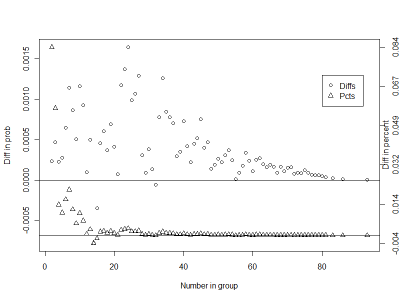# Posts Tagged ‘ matrix ’

## Example 9.21: The birthday "problem" re-examined

February 23, 2012
ByThe so-called birthday paradox or birthday problem is simply the counter-intutitive discovery that the probability of (at least) two people in a group sharing a birthday goes up surprisingly fast as the group size increases. If the group is only 23 peo...

## Once you’re comfortable with 2-arrays and 2-matrices, you…

October 15, 2011
ByOnce you’re comfortable with 2-arrays and 2-matrices, you can move up a dimension or two, to 4-arrays or 4-tensors. You can move up to a 3-array / 3-tensor just by imagining a matrix which “extends back into the blackboard”. Like a 5 × 5 ma...

## Le Monde puzzle [#738]

September 1, 2011
ByThe Friday puzzle in Le Monde this week is about “friendly perfect squares”, namely perfect squares x2__10 and y2__10 with the same number of digits and such that, when drifting all digits of x2 by the same value a (modulo 10), one recovers y2. For instance, 121 is “friend” with 676. Here is my R

## Example 9.1: Scatterplots with binning for large datasets

July 5, 2011
ByScatterplots can get very hard to interpret when displaying large datasets, as points inevitably overplot and can't be individually discerned. A number of approaches have been crafted to help with this problem. One approach uses binning. This approa...

## Summarize Data by Several Variables

May 23, 2011
ByHere's an example how to conveniently summarize data with the cast function (package reshape). By the way you see how this could be done "in-conveniently" by hand. You also see how a for-loop works and how a matrix is constructed and fill...

## Block diagonal matrices in R

April 13, 2011
ByAs far as I can tell, R doesn’t have a function for building block diagonal matrices so as I needed one, I’ve coded it myself. It might save someone some time. Example: Let m1 and m2 two square matrices. Selec … Continue reading →

## Example 7.31: Contour plot of BMI by weight and height

April 5, 2010
ByA contour plot is a simple way to plot a surface in two dimensions. Lines with a constant Z value are plotted on the X-Y plane.Typical uses include weather maps displaying "isobars" (lines of constant pressure), and maps displaying lines of constant e...